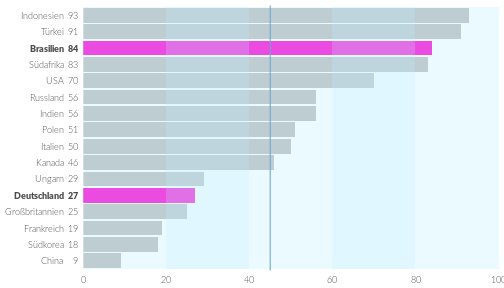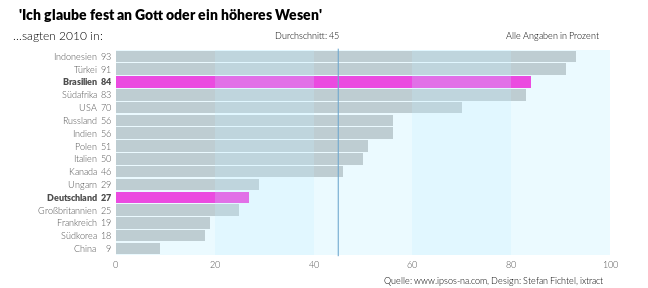A not so simple bar plot example using ggplot2

This is a reproduction of the (simple) bar plot of chapter 6.1.1 in Datendesign mit R with ggplot2. To download the data you can use the following lines:

And to download the original script and the base R version of this plot:

After downloading check out the original pdf version of this plot in `data/beispielcode/pdf/balkendiagramm_einfach.pdf`.

Preparing data

Here are some steps to modify the data such that it can be easily used with ggplot2.

The basic plot

First we add the geoms, then modifications to the scales and flip of the coordinate system. The remaining code is just modifying the appearance.Annotations and layout

Of course you can simply add the title and text annotations to the plot using ggplot2, but I didn’t find a way to do the exact placement comparable to the original version without the package grid.And now we can add the final annotations to the plot: